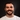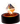Mar 01, 2019 · 2 min

# A basic introduction to functional programming principles in ReasonML

#reasonml
#functionalSeif Ghezala@seifghezala

Functional programming is a programming paradigm based on several principles such as immutability and purity.

ReasonML extends the functional language OCaml and compiles to JavaScript.

This article aims to introduce you to some very basic functional principles present in ReasonML and OCaml. We will see how these principles are closely related and help avoid errors.

### Immutability

#### What is a variable?

A variable is an identifier bound to a specific value.

``````// name is a variable bound to the value "John"
let name = "John";``````

#### Mutable variables

In most programming languages, a variable can be changed to identify another value after its initial declaration:

``````// initial declaration: name identifies "John"
let name = "John";

// mutation: name identifies "Marie" now
name = "Marie";``````

When this happens, we say that the variable is mutated. Therefore, by design, the variable name is mutable.

#### Immutable variables in Reason

Reason approaches variable bindings differently. In fact, a variable always identifies the same value assigned to it during declaration. This prevents variables from being mutated, making most variables in Reason immutable:

``````// initial declaration: name identifies "John"
let name = "John";

// Error
name = "Marie";``````

Note: not all variable types in Reason are immutable. Some variables such as arrays and objects can actually be mutated.

#### Pure and impure functions

A pure function is one that doesn’t produce any effects on its surrounding state.

In multi-paradigms programming languages such as JavaScript, it’s possible to create both pure and impure functions. This makes it possible to achieve the same results through completely different programming styles.

Let’s create a function that counts the number of occurrences of a certain word in a given array:

``````// example 1: impure function
let count = 0;
function countWordOccurences(arr, word) {
for (let value in arr) {
if(value === word) {
count++;
}
}
}

// example 2: pure function
function countWordOccurences(arr, word) {
arr.reduce((count, value) => {
return value === word ? count + 1 : count;
}, 0):
}``````

Both examples achieve the same result.

However, the function in the first example is impure because it alters the value of count , which is in its surrounding state. This is what we call a side effect.

ReasonML is not a pure functional programming language. This means that you can still write impure functions that produce side effects.

However, the immutable bindings we saw earlier will enforce you most of the time to write pure functions. Since the count binding can’t change, you can’t alter its value and reproduce the code of the first example.

### Functions as first-class citizens

When functions are first-class citizens, they are considered just like values. They can be passed around as arguments. If you’re familiar with JavaScript, this concept is probably not surprising for you.

Why is this is useful? Simply because it opens the door to some interesting language features.

#### Higher-order functions

A higher-order function is one that takes a function as an argument or returns one:

``````let numbers = [1, 2, 3, 4];

let multiplyBy2 = x => x * 2;

let numbersX2 = List.map(multiplyBy2, numbers);``````

`List.map` is a higher-order function. It takes two arguments: a function and a list and returns a list which is the result of applying the input function of the entries of the input list:

``````let getMultiplierByN = n => x => n * x;

let multiplierBy2 = getMultiplierByN(2);

multiplierBy2(3); // output: 6``````

`getMultiplierByN` is another higher-order function. It takes an argument n and returns a function that multiplies its argument by n . We used it to create a function that multiples numbers by 2.

#### Closures

Closure is when a function is able to remember and access its lexical scope even when that function is executing outside its lexical scope. Kyle Simpson, You Don’t Know JS

In fact, we used the concept of closure in the last `getMultiplierByN` function example. The function returned by `getMultiplierByN` has access to the value n, which is stored in the scope of its parent function `geMultiplierByN`.

#### Partial function applications and currying

Higher-order functions + closures = curriable functions.

Let’s look at the following example:

``````// function add: takes 2 arguments: x and y and returns their sum
let add = (x, y) => x + y;``````

In Reason, this function is automatically converted to:

``let add = x => y => x + y;``

This is the equivalent in JavaScript:

``````// function add: takes an argument x and returns
// a function F

// function addX: it takes an argument y and returns its sum
// with the value x stored in the closure of add

return x + y;
}
}``````

This allows us to partially call the function:

``````let add5 = add(5);